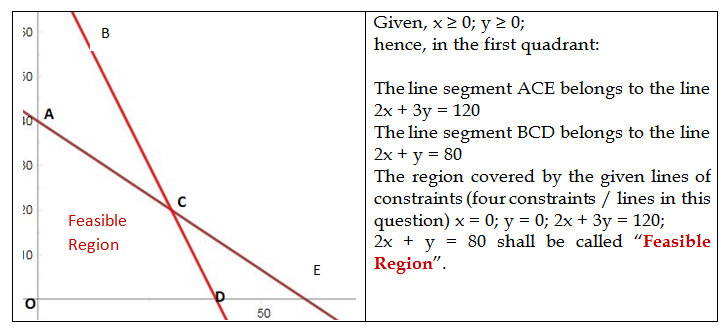top of page

# What is Feasible Region in solving LPP and what are its associated terms?

In my first blog on Linear Programming, you got an idea of Linear Programming and Linear Programming Problems (LPP). In my second blog of the series, you learned about some basic terms of LPP. In the present blog, I move ahead in giving you the beginning of (graphical) technique of solving an LPP, and you must know some more basic terms which are associated with finding of solution of an LPP using graphical method.

We go back to our first problem which I gave in my first blog:

A company manufactures two types of screws: type A and type B. All the screws have to pass through a threading machine and a slotting machine. The box of type A screws requires 4 minutes on the threading machine and 6 minutes on this slotting machine. A box of type B screws requires 6 minutes on the threading machine and 3 minutes on this slotting machine.

In a week, each machine is available for 4 hours on any day. On selling these screws, the company gets a profit of Rs. 7 per box on type A screws and Rs. 10 per box on type B screws. Formulate this problem as a LPP to maximize the profit to the company.

The LPP was formulated in this way:

Let x and y be the numbers of screws of type Aand type B respectively.

Constraints obviously in terms of given parameters:

1. x ≥ 0; y ≥ 0.

2. For type A screws, the threading machine requires 4 min, and slotting machine requires 6 min. 4x + 6y ≤ 240; and, 6x + 3y ≤ 240 {Availability of 4 hours = 240 Minutes}. That is: 2x + 3y ≤ 120; and, 2x + y ≤ 80

Thus we have four straight line inequalities:

x ≥ 0; y ≥ 0; 2x + 3y ≤ 120; 2x + y ≤ 80

{Remember for any LPP, you will always get two inequalities, these are, x ≥ 0; y ≥ 0. The first two inequalities x ≥ 0 and y ≥ 0 speak loudly that our graph will belong to first quarter only in a two-dimensional frame of reference.

By changing these four inequalities in equations, we get: x = 0, y = 0, 2x + 3y = 120 and 2x+y = 80. The graph of these four lines is given below:Thus the feasible region for the given question is: OACDO.

This feasible region is a convex polygon (a convex polygon is defined as a polygon with all its interior angles less than 180°) for a linear programming problem and points O, A, C, D, O are called corner point (or vertex) of the feasible region.

The region other than feasible region is called “Infeasible Region”.

Next, we have to find out the coordinates of all the corner points of feasible region OACDO, i.e., of points O, A, C and D; which are:

O (0,0); A (0,40); C (30,20); D (40,0).

All points within and on the boundary of the feasible region are known as “Feasible Solutions”. Thus there are an infinite number of feasible solutions to the given LPP. All points outside the feasible region, i.e., in infeasible region are known as “Infeasible Solutions”, for example, the point E (60,0) is an infeasible solution to the given LPP.

Finally, an important term “Optimal feasible Solution” and how to get it will be introduced to you. Two more terms “Bounded Region” and “Unbounded Region” are also in the wait line which I shall explain later in this series of blogs on Linear Programming.

Tags:

###### Featured Posts
Check back soon
Once posts are published, you’ll see them here.